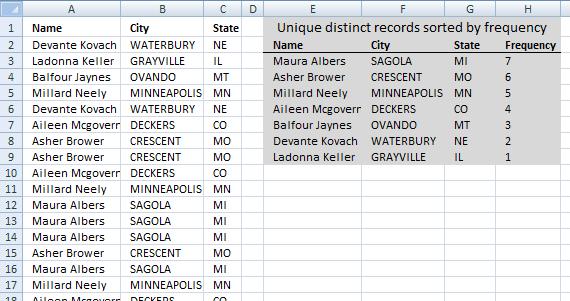Author: Oscar Cronquist Article last updated on August 14, 2019How can you use large with multiple criteria?? Example looking for top 5 of a list based on name, state and city..

The formula entered in cell E3 extracts unique distinct records sorted based on frequency or count.

Array formula in cell E3:

=INDEX(\$A\$2:\$C\$29, MATCH(LARGE(IF(COUNTIFS(\$E\$2:E2, \$A\$2:\$A\$29, \$F\$2:F2, \$B\$2:\$B\$29, \$G\$2:G2, \$C\$2:\$C\$29)=0, COUNTIFS(\$A\$2:\$A\$29, \$A\$2:\$A\$29, \$B\$2:\$B\$29, \$B\$2:\$B\$29, \$C\$2:\$C\$29, \$C\$2:\$C\$29), ""), 1), COUNTIFS(\$A\$2:\$A\$29, \$A\$2:\$A\$29, \$B\$2:\$B\$29, \$B\$2:\$B\$29, \$C\$2:\$C\$29, \$C\$2:\$C\$29)*(COUNTIFS(\$E\$2:E2, \$A\$2:\$A\$29, \$F\$2:F2, \$B\$2:\$B\$29, \$G\$2:G2, \$C\$2:\$C\$29)=0), 0), COLUMN(A1))

#### How to enter an array formula

1. Copy above array formula.
2. Select cell E3
3. Paste array formula to cell E3
4. Press and hold CTRL + SHIFT simultaneously.
5. Press Enter once.
6. Release all keys.

If you did it right the formula is now enclosed with curly brackets, don't enter these characters yourself. They appear automatically if you followed the above steps.

#### How to copy array formula and paste to remaining cells

1.  Select cell E3
2. Copy cell (not formula). (CTRL + c)
3. Select cell range F3:G3
4. Paste to cell range F3:G3. (CTRL + v)
5. Select cell range E3:G3.
6. Copy cells (not formulas). (CTRL + c)
7. Select cell range E4:G9.
8. Paste to cell range E4:G9. (CTRL + v)

Regular formula in cell H3:

=COUNTIFS(\$A\$2:\$A\$29, E3, \$B\$2:\$B\$29, F3, \$C\$2:\$C\$29, G3)

### Explaining array formula in cell E3

#### Step 1 - Identify records not displayed yet

The COUNTIFS function allows you to count cells based on multiple conditions, this makes it ideal for identifying unique distinct records, one condition corresponds to one value in the record.

If you have three values in one record then the COUNTIFS function contains three conditions or six arguments. Each condition has two arguments, the first argument in each pair is a growing cell reference meaning it expands when you copy the cell and paste to cells below.

COUNTIFS(\$E\$2:E2,\$A\$2:\$A\$29,\$F\$2:F2,\$B\$2:\$B\$29,\$G\$2:G2,\$C\$2:\$C\$29)=0

becomes

{0; 0; 0; 0; 0; 0; 0; 0; 0; 0; 0; 0; 0; 0; 0; 0; 0; 0; 0; 0; 0; 0; 0; 0; 0; 0; 0; 0}=0

and returns

{TRUE; TRUE; TRUE; TRUE; TRUE; TRUE; TRUE; TRUE; TRUE; TRUE; TRUE; TRUE; TRUE; TRUE; TRUE; TRUE; TRUE; TRUE; TRUE; TRUE; TRUE; TRUE; TRUE; TRUE; TRUE; TRUE; TRUE; TRUE}.

This makes sense since no values have been displayed yet by the formula in cells above cell E3.

#### Step 2 - Calculate record frequency

The COUNTIFS function also lets you count identical records, by using the IF function we can convert the boolean values to the corresponding count if TRUE and blank "" if FALSE.

IF(COUNTIFS(\$E\$2:E2, \$A\$2:\$A\$29, \$F\$2:F2, \$B\$2:\$B\$29, \$G\$2:G2, \$C\$2:\$C\$29)=0, COUNTIFS(\$A\$2:\$A\$29, \$A\$2:\$A\$29, \$B\$2:\$B\$29, \$B\$2:\$B\$29, \$C\$2:\$C\$29, \$C\$2:\$C\$29), "")

becomes

IF({TRUE; TRUE; TRUE; TRUE; TRUE; TRUE; TRUE; TRUE; TRUE; TRUE; TRUE; TRUE; TRUE; TRUE; TRUE; TRUE; TRUE; TRUE; TRUE; TRUE; TRUE; TRUE; TRUE; TRUE; TRUE; TRUE; TRUE; TRUE}, COUNTIFS(\$A\$2:\$A\$29, \$A\$2:\$A\$29, \$B\$2:\$B\$29, \$B\$2:\$B\$29, \$C\$2:\$C\$29, \$C\$2:\$C\$29), "")

becomes

IF({TRUE; TRUE; TRUE; TRUE; TRUE; TRUE; TRUE; TRUE; TRUE; TRUE; TRUE; TRUE; TRUE; TRUE; TRUE; TRUE; TRUE; TRUE; TRUE; TRUE; TRUE; TRUE; TRUE; TRUE; TRUE; TRUE; TRUE; TRUE}, {2; 1; 3; 5; 2; 4; 6; 6; 4; 5; 7; 7; 7; 6; 7; 5; 4; 7; 7; 6; 4; 7; 3; 6; 5; 6; 3; 5}, "")

and returns

{2; 1; 3; 5; 2; 4; 6; 6; 4; 5; 7; 7; 7; 6; 7; 5; 4; 7; 7; 6; 4; 7; 3; 6; 5; 6; 3; 5}.

The first value in the array above corresponds to the first record (row) in cell range \$A\$2:\$C\$29, thus 2 tells us that there is an identical record somewhere in cell range \$A\$2:\$C\$29. If we examine the list above you can see that the first record (row 2) is identical to the record in row 6.

Row 6 is the fifth value in the array above, that value must also be 2 if the formula works as intended. Yes, the fifth value is also 2.

#### Step 3 - Extract largest number from array

The LARGE function lets you extract the k-th largest value in a cell range or array, in this case, we are looking for the largest value and k is therefore 1.

LARGE(IF(COUNTIFS(\$E\$2:E2, \$A\$2:\$A\$29, \$F\$2:F2, \$B\$2:\$B\$29, \$G\$2:G2, \$C\$2:\$C\$29)=0, COUNTIFS(\$A\$2:\$A\$29, \$A\$2:\$A\$29, \$B\$2:\$B\$29, \$B\$2:\$B\$29, \$C\$2:\$C\$29, \$C\$2:\$C\$29), ""), 1)

becomes

LARGE({2; 1; 3; 5; 2; 4; 6; 6; 4; 5; 7; 7; 7; 6; 7; 5; 4; 7; 7; 6; 4; 7; 3; 6; 5; 6; 3; 5},1)

and returns 7.

#### Step 4 - Find position of largest value

The MATCH function returns the relative position of a value in an array or cell range. The array we match against is the frequency numbers multiplied with an array with boolean values that indicates if a record has already been shown in cells above the current cell.

MATCH(LARGE(IF(COUNTIFS(\$E\$2:E2,\$A\$2:\$A\$29,\$F\$2:F2,\$B\$2:\$B\$29,\$G\$2:G2,\$C\$2:\$C\$29)=0,COUNTIFS(\$A\$2:\$A\$29,\$A\$2:\$A\$29,\$B\$2:\$B\$29,\$B\$2:\$B\$29,\$C\$2:\$C\$29,\$C\$2:\$C\$29),""),1),COUNTIFS(\$A\$2:\$A\$29,\$A\$2:\$A\$29,\$B\$2:\$B\$29,\$B\$2:\$B\$29,\$C\$2:\$C\$29,\$C\$2:\$C\$29)*(COUNTIFS(\$E\$2:E2,\$A\$2:\$A\$29,\$F\$2:F2,\$B\$2:\$B\$29,\$G\$2:G2,\$C\$2:\$C\$29)=0),0)

becomes

MATCH(7,COUNTIFS(\$A\$2:\$A\$29,\$A\$2:\$A\$29,\$B\$2:\$B\$29,\$B\$2:\$B\$29,\$C\$2:\$C\$29,\$C\$2:\$C\$29)*(COUNTIFS(\$E\$2:E2,\$A\$2:\$A\$29,\$F\$2:F2,\$B\$2:\$B\$29,\$G\$2:G2,\$C\$2:\$C\$29)=0),0)

becomes

MATCH(7,{2; 1; 3; 5; 2; 4; 6; 6; 4; 5; 7; 7; 7; 6; 7; 5; 4; 7; 7; 6; 4; 7; 3; 6; 5; 6; 3; 5}*(COUNTIFS(\$E\$2:E2,\$A\$2:\$A\$29,\$F\$2:F2,\$B\$2:\$B\$29,\$G\$2:G2,\$C\$2:\$C\$29)=0),0)

becomes

MATCH(7,{2; 1; 3; 5; 2; 4; 6; 6; 4; 5; 7; 7; 7; 6; 7; 5; 4; 7; 7; 6; 4; 7; 3; 6; 5; 6; 3; 5}*{TRUE; TRUE; TRUE; TRUE; TRUE; TRUE; TRUE; TRUE; TRUE; TRUE; TRUE; TRUE; TRUE; TRUE; TRUE; TRUE; TRUE; TRUE; TRUE; TRUE; TRUE; TRUE; TRUE; TRUE; TRUE; TRUE; TRUE; TRUE},0)

becomes

MATCH(7,{2; 1; 3; 5; 2; 4; 6; 6; 4; 5; 7; 7; 7; 6; 7; 5; 4; 7; 7; 6; 4; 7; 3; 6; 5; 6; 3; 5},0)

and returns 11. Number 7 is found int the 11-th position in the array.

#### Step 5 - Return value based on position

INDEX(\$A\$2:\$C\$29,MATCH(LARGE(IF(COUNTIFS(\$E\$2:E2,\$A\$2:\$A\$29,\$F\$2:F2,\$B\$2:\$B\$29,\$G\$2:G2,\$C\$2:\$C\$29)=0,COUNTIFS(\$A\$2:\$A\$29,\$A\$2:\$A\$29,\$B\$2:\$B\$29,\$B\$2:\$B\$29,\$C\$2:\$C\$29,\$C\$2:\$C\$29),""),1),COUNTIFS(\$A\$2:\$A\$29,\$A\$2:\$A\$29,\$B\$2:\$B\$29,\$B\$2:\$B\$29,\$C\$2:\$C\$29,\$C\$2:\$C\$29)*(COUNTIFS(\$E\$2:E2,\$A\$2:\$A\$29,\$F\$2:F2,\$B\$2:\$B\$29,\$G\$2:G2,\$C\$2:\$C\$29)=0),0),COLUMN(A1))

becomes

INDEX(\$A\$2:\$C\$29,11,COLUMN(A1))

becomes

INDEX(\$A\$2:\$C\$29, 11, 1)

and returns "Maura Albers" in cell E3.